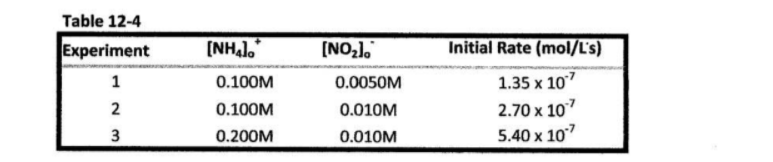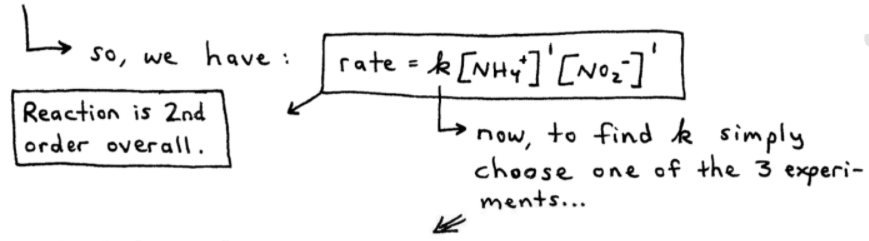# S12E3 - Method of Initial Rates

## The Form of the Rate Law is Determined Experimentally

To calculate the form of the rate law, we use the Method of Initial Rates.

### Method of Initial Rates

ex:  Consider the reaction below,

NH4+(aq)  +  NO2-(aq)  →  N2(g)  +  2H2O(l)

So,  rate  =  - Δ [NH4+] / Δt  =  k [NH4+]n [NO2-]m

We need to solve for n, m, and k.  Here's the above information again, in an easier-to-follow handwritten format:To solve for n, m, and then k, we will use the data from 3 experiments in Table 12-4 below:-----

FIRST - Calculating n and m, in:
rate  =  k [NH4+]n [NO2-]m

- ratio of rates 1 and 2:  the image below shows how we can use experiments 1 and 2 data from Table 12-4.  This allows us to isolate and solve for "m"

- ratio of rates 2 and 3:  the image below shows how we can use experiments 2 and 3 data from Table 12-4.  This allows us to isolate and solve for "n"As you can see above, we've chosen ratios such that only n or m remains unknown, due to one of them "cancelling out."

So, n = 1 and m = 1, and the reaction is 2nd order overall:

rate  =  k [NH4+]1 [NO2-]1### Calculating the Rate Constant, k

➞ NEXT - Calculating the rate constant k:  we can use any of the 3 experiments to find k.

Using data from Experiment 1 in Table 12-4, we get:

rate  =  k [NH4+]1 [NO2-]1

1.35 x 10-7 mol / L.s  =  k (0.100M)1 (0.0050M)1

1.35 x 10-7 mol / L.s  =  k (0.00050M2)

0.00027 L / mol.s  =  k

When solving for k, the units for k are the most difficult part.  So be careful.

=====

### Determining a Differential Rate Law

Remember, a "differential rate law" expresses a reaction rate as a function of reactant concentrations.

ex:  Using the data listed below in Table 12-5, calculate the order for each reactant, the overall reaction order, and the value of the rate constant, for the following reaction:

BrO3-  +  5Br-  +  6H+  ⟶  3Br2  +  3H2OWe cannot use the coefficients of the balanced reaction as our "orders" for each reactant, so we have:

rate  =  k [BrO3-]n [Br-]m [H+]p

To solve for n, choose experiments 1 and 2 in Table 12-5 because this enables "m" and "p" unknowns to drop out.  See here...Similarly, we can solve for m and p by choosing the appropriate experiments:

➞ to solve for m, divide:  rate 3 / rate 2
➞ to solve for p, divide:  rate 4 / rate 1=====

Our next topic in SECTION 12 - Chemical Kinetics,

We'll discuss all there is to know about the First Order, Second Order, and Zero Order Integrated Rate Laws...